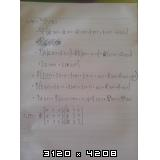# Angular momentum operators on a wave function

## Homework Statement

Particle is in a state with wave function $\psi (r) = A z (x+y)e^{-\lambda r}.$
a) What is the probability that the result of the $L_z$ measurement is 0?
b) What are possilble results and what are their probabilities of a $L^2$ measurement?
c) What are possilble results and what are their probabilities of a $L_x$ measurement?

## The Attempt at a Solution

Firstly, I tried to write wave function in spherical harmonics form:
\begin{align*} \psi (r) &= \alpha z (x+y); \ \ \alpha= A e^{-\lambda r}\\ &= \alpha r \cos\theta (r \sin\theta\cos\phi+r \sin\theta \sin \phi)\\ &= \alpha r^2 \cos\theta \sin \theta (\cos\phi + \sin \phi) \\ &=\alpha r^2\cos\theta \sin\theta((\frac{1}{2}+\frac{i}{2})e^{-i \phi}+(\frac{1}{2}-\frac{i}{2})e^{i \phi}) \\ &= -{\alpha \over 2} r^2 \sqrt{\frac{8 \pi}{15}} ((1+i)Y_{2,-1}+(1-i)Y_{2,1}) \end{align*}

Then, I normalized this function:
$$|\psi,r\rangle = {\alpha \over {2c}} r^2 \sqrt{\frac{8 \pi}{15}} ((1+i) c Y_{2,-1}+(1-i) c Y_{2,1}) \\ \Longrightarrow c^2 |(1+i)|^2+c^2 |(1-i)|^2=1 \\ c={1 \over 2}$$
So finally, I get my wave function:
$$\psi (r) = \alpha r^2 \sqrt{\frac{8 \pi}{15}} ({1 \over 2}(1+i) Y_{2,-1}+{1 \over 2}(1-i)Y_{2,1})$$
Can someone check this normalization, I am not sure if I did it correctly?

a) Form definition $L_z|l,m\rangle=\hbar m |l,m\rangle$ I can't get 0 as a result, because there isn't any element of a wave function with m=0. So the answer is 0 probability.

b) Form definition $L^2|l,m\rangle=\hbar^2 l(l+1) |l,m\rangle$ I can only get the result $6 \hbar^2$ with 100% probability, since $l$ is in both elements of a wave function 2.

c) Form definition $L_x|l,m\rangle=\frac{L_+ + L_-}{2} |l,m\rangle$, I get:
\begin{align*} L_+ |2,1\rangle &= 2 \hbar |2,2\rangle \\ L_+ |2,-1\rangle &= \sqrt{6} \hbar |2,0\rangle \\ L_- |2,1\rangle &= \sqrt{6} \hbar |2,0\rangle \\ L_- |2,-1\rangle &= 2 \hbar |2,-2\rangle\\ \end{align*}
\begin{align*} \Longrightarrow L_x |2,1\rangle &= \hbar (|2,2\rangle + \frac{\sqrt{6}}{2} |2,0\rangle)\\ \Longrightarrow L_x |2,-1\rangle &= \hbar (|2,-2\rangle + \frac{\sqrt{6}}{2} |2,0\rangle) \end{align*}

So the possible results are $\hbar$ and $\frac{\sqrt{6}}{2} \hbar$? But how can I now find their probabilities? I tried to write:
$$L_x |\psi\rangle = \sqrt{\frac{8 \pi}{15}} \alpha \hbar r^2(\frac{1+i}{2}(|2,2\rangle+{\sqrt{6} \over2} |2,0\rangle)+\frac{1-i}{2}(|2,-2\rangle +{\sqrt{6} \over2} |2,0\rangle)),$$
but the squares of absolute values of coefficients are above 1.

DrClaude
Mentor
\begin{align*} \psi (r) &= \alpha z (x+y); \ \ \alpha= A e^{-\lambda r}\\ &= \alpha r \cos\theta (r \sin\theta\cos\phi+r \sin\theta \sin \phi)\\ &= \alpha r^2 \cos\theta \sin \theta (\cos\phi + \sin \phi) \\ &=\alpha r^2\cos\theta \sin\theta((\frac{1}{2}+\frac{i}{2})e^{-i \phi}+(\frac{1}{2}-\frac{i}{2})e^{i \phi}) \\ &= -{\alpha \over 2} r^2 \sqrt{\frac{8 \pi}{15}} ((1+i)Y_{2,-1}+(1-i)Y_{2,1}) \end{align*}

Then, I normalized this function:
$$|\psi,r\rangle = {\alpha \over {2c}} r^2 \sqrt{\frac{8 \pi}{15}} ((1+i) c Y_{2,-1}+(1-i) c Y_{2,1}) \\ \Longrightarrow c^2 |(1+i)|^2+c^2 |(1-i)|^2=1 \\ c={1 \over 2}$$
So finally, I get my wave function:
$$\psi (r) = \alpha r^2 \sqrt{\frac{8 \pi}{15}} ({1 \over 2}(1+i) Y_{2,-1}+{1 \over 2}(1-i)Y_{2,1})$$
Can someone check this normalization, I am not sure if I did it correctly?
I don't understand what you did here. Actually, compare that last line with the last line above; do you see any difference? Also, what about ##\alpha## (and the ##A## it contains)?

•gasar8
Aha, I forgot that $Y_l^{-m}=(-1)^m Y_l^m$, so I get:
$$|\psi\rangle = {\alpha \over 2} r^2 \sqrt{\frac{8 \pi}{15}} \big((1+i)Y_2^{-1}-(1-i)Y_2^{1}\big)$$

Ok, here I tried something we did at lectures, but apparently I didn't understand it well. So if I go the long way:
$$\langle \psi,r | \psi,r \rangle = \frac{A A^\ast e^{-2\lambda r}}{4} {8\pi \over 15}\bigg[ \big((1-i) \langle 2, -1| - (1+i)\langle 2,1|)((1+i)|2,1\rangle-(1-i)|2,1\rangle \big) \bigg] =1\\ \frac{2 A A^\ast \pi}{15}e^{-2\lambda r} (2+2) = 1\\ |A|=\sqrt{\frac{15}{8 \pi}}e^{-\lambda r}$$
So I get my wave function:
$$|\psi\rangle={r^2 \over 2} \bigg[(1+i) Y_2^{-1}-(1-i)Y_2^1\bigg].$$

EDIT: Forgot square root over fraction in $|A|$.

Last edited:
I've got one similar exercise, but instead of angular momentum I've got spins. And I've got the same problems at $S_x$ measurements.
So the exercise goes:
We have got two particles with $S_1=1$ and $S_2=1$. We know that $S_{1z}|\psi_1\rangle=\hbar |\psi_1\rangle$ and $S_{2x}|\psi_2\rangle = \hbar |\psi_2\rangle.$

a) Find wave function $|\psi_1\rangle$ in $S_{1z}$ basis and $|\psi_2\rangle$ in $S_{2z}$ basis.
b) We measure $S^2$ of total spin. What are possible outcomes and what are their probabilities?
c) Find expectation value and uncertainty of $S^2$.
d) We measure x component of total spin. What are possible outcomes and what are their probabilities?

a) $|\psi_1\rangle = |11\rangle \\ |\psi_2\rangle = {1 \over 2} |1-1\rangle + {1 \over \sqrt{2}} |10\rangle+ {1 \over 2} |11\rangle.$ Can someone just check this?
b)\begin{align*} |\psi_{12}\rangle&={1 \over 2}|1\rangle|-1\rangle+{1 \over \sqrt{2}} |1\rangle|0\rangle+{1 \over 2}|1\rangle|1\rangle=\\ &={1 \over \sqrt{24}}|20\rangle+{1 \over \sqrt{12}}|00\rangle+{1 \over 2}|21\rangle+{1 \over 2}|11\rangle+{1 \over 2}|22\rangle \end{align*}
For $S^2|\psi_{12}\rangle=\hbar^2 s(s+1)|\psi_{12}\rangle,$ we get:
\begin{align*} &Results \ \ \ \ &Probability\\ &6\hbar^2 &{13\over24}\\ &2\hbar^2 &{3 \over 8}\\ &0 &{1 \over 12} \end{align*}

c) Expectation value is $\langle S^2 \rangle = \langle \psi|S^2|\psi\rangle=4\hbar^2,$ but I can't find uncertainty? I am thinking in this way:
$$\delta_{S^2}=\sqrt{\langle S^2\rangle- \langle S \rangle ^2} or \\ \delta_{S^2}=\sqrt{\langle S^4\rangle- \langle S^2 \rangle ^2}?$$

d) Now, same problem as at the angular momentum. How do I find outcomes and probabilities? I tried with $S_x=\frac{S_++S_-}{2}$, but got some weird result, from which I can't find anything (As mentioned - like at angular momentum in previous post). Then I was thinking about Pauli matrices, so that possible outcomes would be $\pm {\hbar \over 2}$, but how can I apply this matrix to my wavefunction of 1x1 spins. I found something on wiki for such spins and tried but got nothing... (This two attempts are in my handwriting downthere):